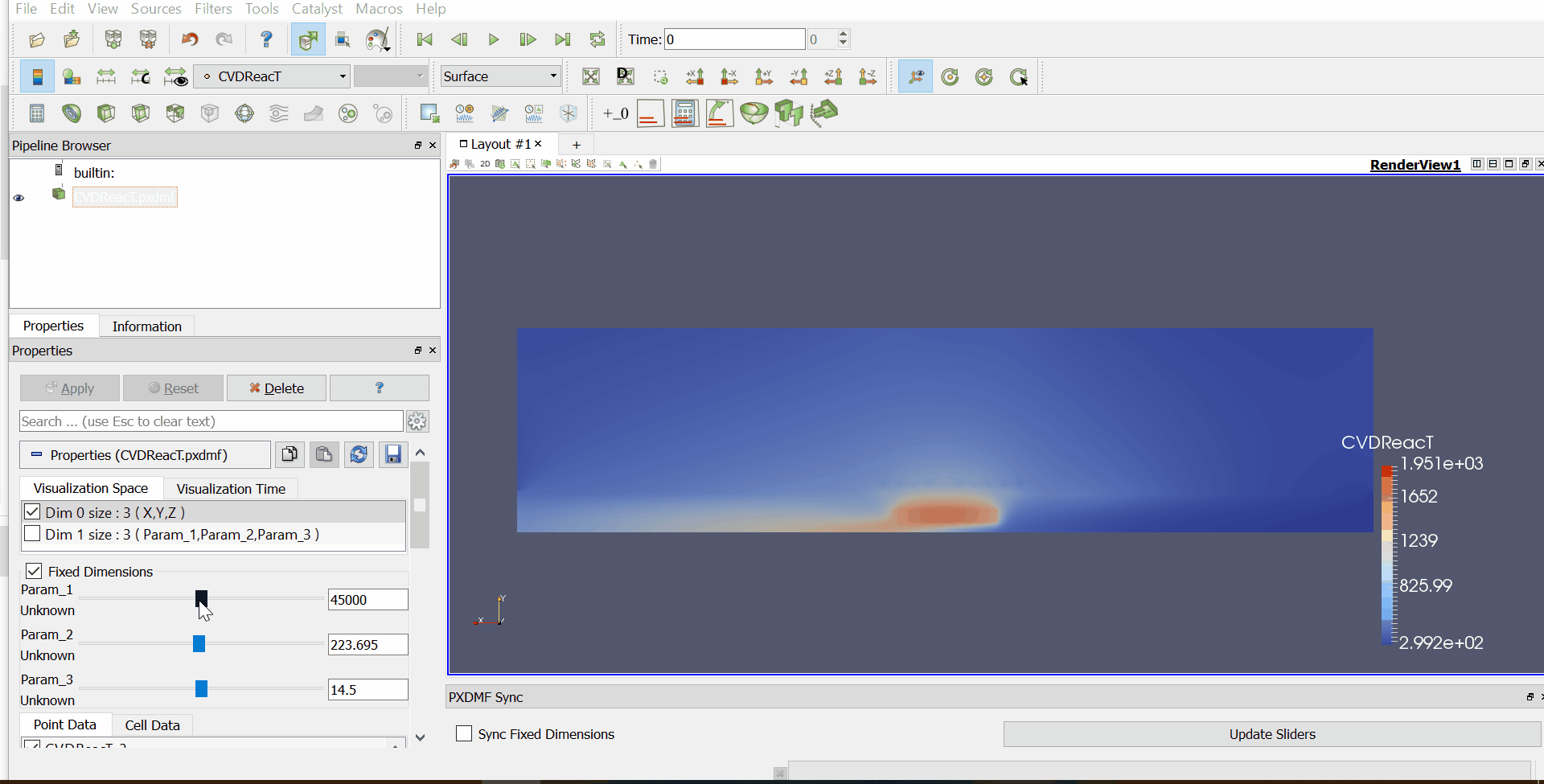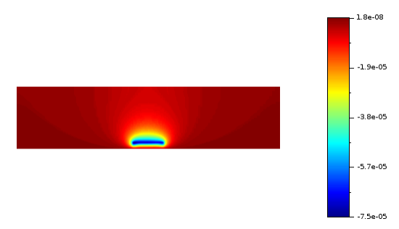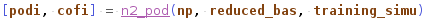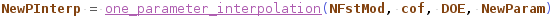# Model reduction on Chemical Vapor Deposition reactorThis paper will show you how to properly use the Scilab Model Reduction toolbox on the case of a Danielson reactor model: a CVD reactor using RF coil to inductively heat the substrate. This CFD study has been run at 69 sparse grid points for 3 varying parameters : Frequency (20 – 70kHz), RF current in coils (150 – 300 A) and Flow (0 – 29 slm). Let see how behaves the imaginary part of the static potential in the cavity and get more informations on non simulated parameters case. It is a steady case.

Nota : Make sure you got the toolbox documentation before starting this tutorial (PDF)

## Case tree

This case is structured as described on the image on the left. You first get your simulation data :

1. Your mesh description with X, Y, Z and Connectivity as text files. The first one gathers X, Y nodes coordinates, one column for each. The second one is the cell description (connectivity) of your mesh. This is an array where the nthline is composed of the position of each nodes (regarding the first coordinates file) composing the nth cell
2. Your field data (text files again) for your DOE simulations, here located in the SimData/Az_i directory for the imaginary part of the static potential.

In order to make it easier, you also got all those imported data, mesh and DOE as Scilab data file in the PODData directory. Finally all the scripts you will need are located in the Scriptsdirectory.

## Data import

As said, data are already available as a Scilab data format. But, if you want to import your own data, you can use the following and provided scripts.

This one allows you to transform text files as double arrays in order to create your Mesh object with the POD toolbox create_mesh function. Be careful, your elem array that gathers the nodal composition of cells have to start index at 1.

This one allows you to transform text files as arrays lists in order to create your simulation object. Each element of your list should correspond to one simulation case for a specific set of parameters.

## Create simulation

Your data and mesh are now saved. It’s time to load them in order to create your learning Simulation object. You will find all the details in the mainPOD.sce script.Thanks to the create_simulation function, you will be able to create a simulation object, depending on the mesh object you just created and the nodal values of your field.

Your data and mesh are now saved. It’s time to load them in order to create your learning Simulation object. You will find all the details in the mainPOD.sce script.Thanks to the create_simulation function, you will be able to create a simulation object, depending on the mesh object you just created and the nodal values of your field.

If you want to check that your import phase (mesh + data) went right, you can also see your simulation thanks to the show_simulation function. Depending on the number of frames (for unsteady cases) you have, you can display a single frame or a complete animation.Az_i field for 45000 Hz / 294.3 A / 14.5 slm

Note that we have defined a limit_conditions object in our script. As said in the POD documentation, you have to take care of your boundary conditions for equation model reduction. But this case will be about a less intrusive reduction method. Still, you have to define a limit_conditions object.

## Generate POD basis

What you get from now : A mesh, a learning simulation.

The idea behind POD is to sum up your system behavior into a few of the most energetic spatial (classical method) or temporal (snapshots method) correlations called modes. Theoretically, those modes constitute an orthonormal basis of your simulation domain. Those modes are sorted in order for you to consider only the more energetic one, and thus reduce your problem complexity. Let see how to generate this modal basis in Scilab.

12 first eigenvalues and associated modes for Az_i field

To do so, you will just have to use the two following function. Note that those functions allow you to generate Scilab objects, with properties such as eigenvectors, eigenvalue, you can access whenever you want.

• The basis generation is made through the compute_pod_basis functions. It could take a bit time because of the construction/diagonalization of a huge correlation matrix. For now, a 100.000 nodes mesh, 1000 frames/snapshots takes 12min computing on Scilab 6 to get the pod_basis.
• Now that you get your pod_basis, you can reduce it keeping only the most energetic modes and visualize them thanks to the reduce_pod_basis function.

Be careful ! As said earlier, the pod basis is theoretically an orthonormal basis, meaning that keeping the full basis will give you the exact solution you would have simulated. But, due to some numerical error, taking too much modes into account increases the reconstruction/prediction error. Take the time to look for the optimal number of modes regarding accuracy vs computing time.

## Data compression

I you want to evaluate the accuracy of your reduced basis, or may be compress your data, try to project your simulation on this new basis.

Simulation (n°69) reconstruction and reconstruction error (n°1 to n°69) considering only 12 modes

To do so you can generate a projected simulation object thanks to the reconstruct_simulationfunction as defined below.

The compute_rel_error function will then return the normalized root mean square deviation between your nodal value of field  and the projected simulation nodes values you get at the previous stage.

On the above image, you can see the reconstruction of the Az_i field for the 69th parametric case and, next to it, the relative error computed for all the parametric cases. For 12 modes, the maximum error reach 4%.

## Parametric prediction

What you get from now : A mesh, a learning simulation, a reduced pod basis.

The idea is that, with a few simulations on specific parameters, you can sum up the behavior of your system in any conditions. This can take a Design of Experiment to set up relevant simulation cases. But once this is done, and the associated reduced basis generated following the previous process, you can now predict your system behavior on non-simulated cases.

A first method could be to project your model equations on the POD basis. But in that case we will leverage a less intrusive method. Practically it consists in interpolating the projection coefficients following specific methods.

Our case is a steady case that varies following a set of 3 parameters : Frequency, RF current and Flow. You can find the entire DOE in the 69EvaluationPoints excel file.

• Thanks to the RBF_interpolation function, you will first compute the projection coefficients on the reduced basis for all of your learning simulations and will interpolate the coefficients for the new set of parameters with Radial Basis Function, based on your DOE (one parameter per column).
• Then you just have to reconstruct your simulation object based on the new coefficients you got and the POD basis, with the predict_simulation function.

Want to see your prediction ? Just use show_simulation function!

Some cases could be unsteady cases. However, unsteady simulations are more complex to predict because we can’t make a simple interpolation on the coefficients, considering that those are time- and parameter-dependent. So you will have to make a second POD on the reduced basis you got, using n2_pod function.

Some cases could be unsteady cases. However, unsteady simulations are more complex to predict because we can’t make a simple interpolation on the coefficients, considering that those are time- and parameter-dependent. So you will have to make a second POD on the reduced basis you got, using n2_pod function.Giving the number of learning parameters, the first reduced_basis and the learning simulation, this function returns a new POD basis and the associated coefficients. Those coefficients are used for the interpolation in the following function :Just as RBF_interpolation, this function will return the interpolated coefficients for the new value of parameters, given the parameter space grid (DOE – have to be line vector), the number of modes n_pod in the first reduced basis and the coefficients given by the n2_podfunction.

Coefficients interpolation based on a 4 points DOE only – plotted by one_parameter_interpolation

And here you are again with your new set of interpolated coefficients.

## Conclusion

Thanks to this tutorial you are now able to leverage the first few features of the Scilab Model Reduction Toolbox in order to generate a Proper Orthogonal Decomposition.